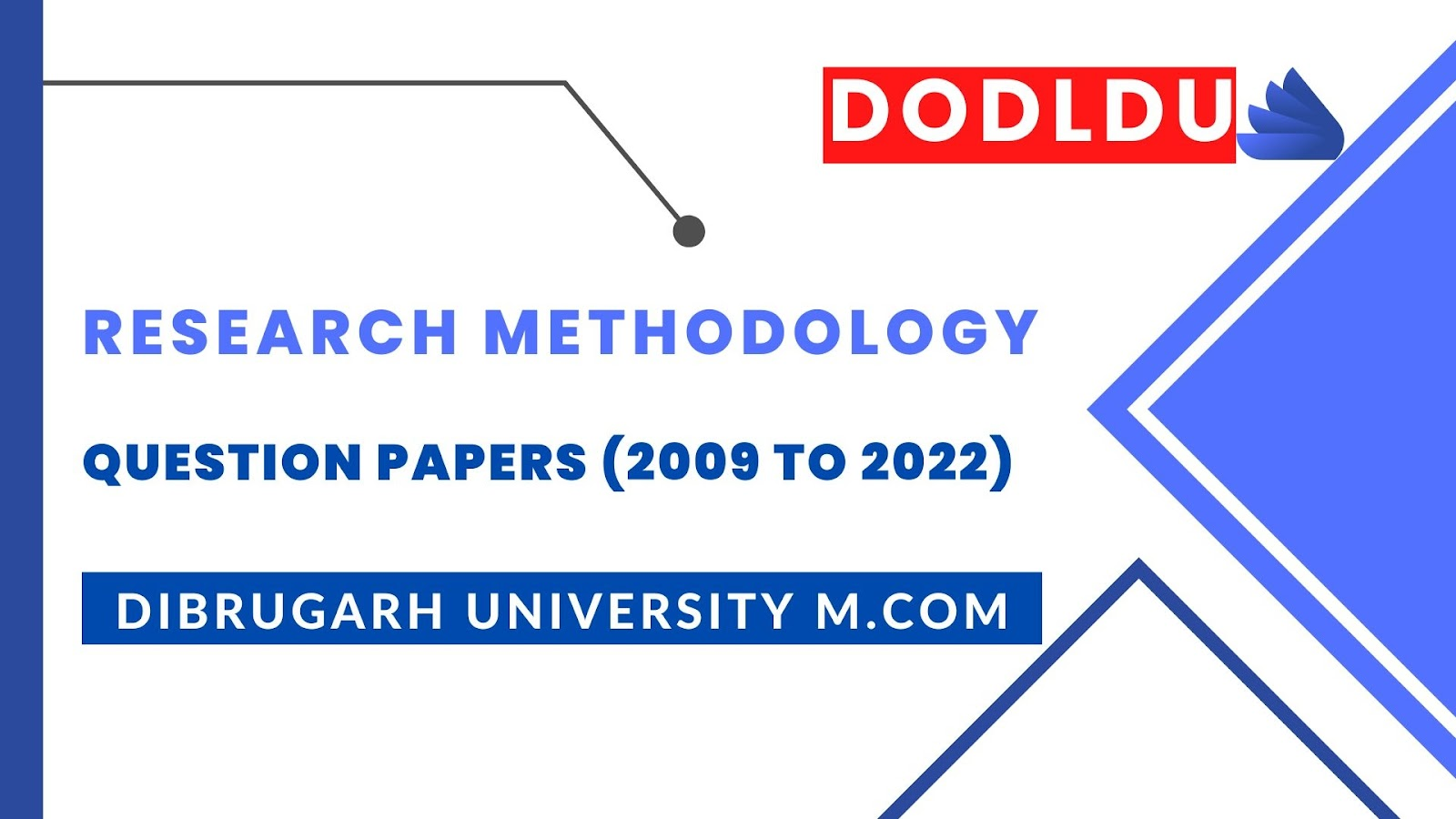# Research Methodology Question Paper 2018 [Dibrugarh University DODLDU M.Com Final Year]

## Research Methodology Question Paper 2018 (Nov-Dec)[Dibrugarh University DODLDU M.Com Final Year]

COMMERCE
Paper: 203
(Research Methodology and Statistical Analysis)
Full Marks: 80
Time: 3 hours
The figures in the margin indicate full marks for the questions
1. (a) What are the different types of research? Explain them in brief.      4+12=16
Or
(b) What do you mean by data? Distinguish between primary data and secondary data.  4+12=16
2. (a) What is measures of skewness? Explain the three different formulas to compute the coefficient of skewness. 6+10=16
Or
(b) What are the different measures of central tendency? How these measures are used in analyzing data under different circumstances?                 4+12=16
3. (a) Explain what is probability in statistics. State the situation under which multiplicative probability model is used. 4+12=16
Or
(b) State ‘Bayes’ theorem and illustrate it with an example.         8+8=16
4. (a) Discuss the methods of determining sample size.                  16
Or
(b) Distinguish between type-I error and type-II Error.              16
5. (a) What is non-parametric statistical test? Under what circumstances non-parametric tests are used?                  6+10=16
Or
(b) Explain Wilcoxon Signed test with appropriate examples.          16
***